# AP Statistics Multiple-Choice Practice Questions: Summarizing Distributions 1

### Test Information11 questions25 minutes

1. The graph below shows household income in Laguna Woods, California.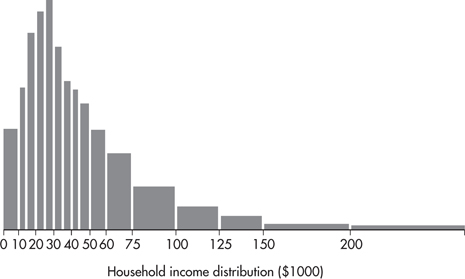What can be said about the ratio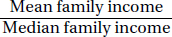?

2. Students in AP Statistics class were timed in seconds while solving a series of mathematical brainteasers. One student's time had a standardized score of z = 2.40. If the times are all changed to minutes, what will then be the student's standardized score?

3. Dieticians are concerned about sugar consumption in teenagers' diets (a 12-ounce can of soft drink typically has 10 teaspoons of sugar). In a random sample of 55 students, the number of teaspoons of sugar consumed for each student on a randomly selected day is tabulated. Summary statistics are noted below: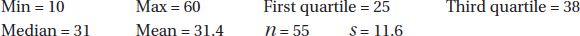Which of the following is a true statement?

4. Problem below refers to the following five boxplots.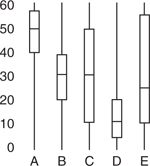To which of the above boxplots does the following histogram correspond?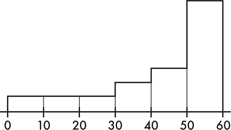5. Problem below refers to the following five boxplots.To which of the above boxplots does the following histogram correspond?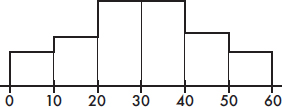6. Problem below refers to the following five boxplots.To which of the above boxplots does the following histogram correspond?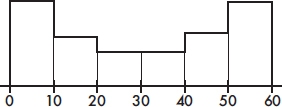7. Problem below refers to the following five histograms: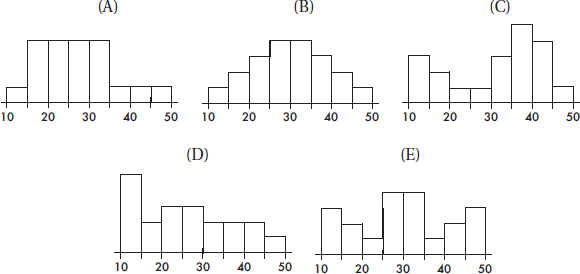To which of the above histograms does the following boxplot correspond?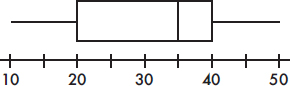8. Problem below refers to the following five histograms:To which of the above histograms does the following boxplot correspond?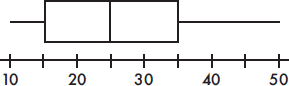9. Problem below refers to the following five histograms:To which of the above histograms does the following boxplot correspond?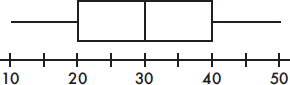10. Below is a boxplot of CO2 levels (in grams per kilometer) for a sampling of 2008 vehicles.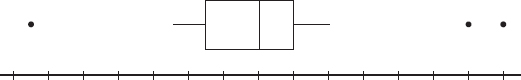Suppose follow-up testing determines that the low outlier should be 10 grams per kilometer less and the two high outliers should each be 5 grams per kilometer greater. What effect, if any, will these changes have on the mean and median CO2 levels?

11. Below is a boxplot of yearly tuition and fees of all four year colleges and universities in a Western state. The low outlier is from a private university that gives full scholarships to all accepted students, while the high outlier is from a private college catering to the very rich.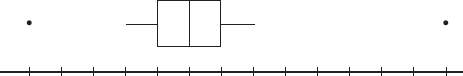Removing both outliers will effect what changes, if any, on the mean and median costs for this state's four-year institutions of higher learning?